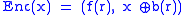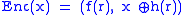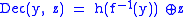xProbabilistic encryptionEncyclopedia
Probabilistic encryption is the use of randomness
Randomness
Randomness has somewhat differing meanings as used in various fields. It also has common meanings which are connected to the notion of predictability of events....

in an encryption
Encryption
In cryptography, encryption is the process of transforming information using an algorithm to make it unreadable to anyone except those possessing special knowledge, usually referred to as a key. The result of the process is encrypted information...

algorithm, so that when encrypting the same message several times it will, in general, yield different ciphertext
Ciphertext
In cryptography, ciphertext is the result of encryption performed on plaintext using an algorithm, called a cipher. Ciphertext is also known as encrypted or encoded information because it contains a form of the original plaintext that is unreadable by a human or computer without the proper cipher...

s. The term "probabilistic encryption" is typically used in reference to public key encryption algorithms, however various symmetric key encryption algorithms achieve a similar property (e.g., block cipher
Block cipher
In cryptography, a block cipher is a symmetric key cipher operating on fixed-length groups of bits, called blocks, with an unvarying transformation. A block cipher encryption algorithm might take a 128-bit block of plaintext as input, and output a corresponding 128-bit block of ciphertext...

s when used in a chaining mode such as CBC). To be semantically secure
Semantic security
Semantic security is a widely used definition for security in an asymmetric key encryption algorithm. For a cryptosystem to be semantically secure, it must be infeasible for a computationally bounded adversary to derive significant information about a message when given only its ciphertext and...

, that is, to hide even partial information about the plaintext
Plaintext
In cryptography, plaintext is information a sender wishes to transmit to a receiver. Cleartext is often used as a synonym. Before the computer era, plaintext most commonly meant message text in the language of the communicating parties....

, an encryption algorithm must be probabilistic
Randomized algorithm
A randomized algorithm is an algorithm which employs a degree of randomness as part of its logic. The algorithm typically uses uniformly random bits as an auxiliary input to guide its behavior, in the hope of achieving good performance in the "average case" over all possible choices of random bits...

.

Probabilistic encryption is particularly important when using public key cryptography. Suppose that the adversary
In cryptography, an adversary is a malicious entity whose aim is to prevent the users of the cryptosystem from achieving their goal...

observes a ciphertext, and suspects that the plaintext is either "YES" or "NO", or has a hunch that the plaintext might be "ATTACK AT CALAIS". When a deterministic encryption
Deterministic encryption
A deterministic encryption scheme is a cryptosystem which always produces the same ciphertext for a given plaintext and key, even over separate executions of the encryption algorithm...

algorithm is used, the adversary can simply try encrypting each of his guesses under the recipient's public key, and compare each result to the target ciphertext. To combat this attack, public key encryption schemes must incorporate an element of randomness, ensuring that each plaintext maps into one of a large number of possible ciphertexts.

An intuitive approach to converting a deterministic encryption scheme into a probabilistic one is to simply pad the plaintext with a random string before encrypting with the deterministic algorithm
Deterministic algorithm
In computer science, a deterministic algorithm is an algorithm which, in informal terms, behaves predictably. Given a particular input, it will always produce the same output, and the underlying machine will always pass through the same sequence of states...

. Conversely, decryption involves applying a deterministic algorithm and ignoring the random padding. However, early schemes which applied this naive approach were broken due to limitations in some deterministic encryption schemes. Techniques such as OAEP
In cryptography, Optimal Asymmetric Encryption Padding is a padding scheme often used together with RSA encryption. OAEP was introduced by Bellare and Rogaway....

integrate random padding in a manner that is secure using any trapdoor permutation.

The first provably-secure probabilistic public-key encryption scheme was proposed by Shafi Goldwasser
Shafi Goldwasser
Shafrira Goldwasser is the RSA Professor of electrical engineering and computer science at MIT, and a professor of mathematical sciences at the Weizmann Institute of Science, Israel.-Biography:...

and Silvio Micali
Silvio Micali
Silvio Micali is an Italian-born computer scientist at MIT Computer Science and Artificial Intelligence Laboratory and a professor of computer science in MIT's Department of Electrical Engineering and Computer Science since 1983. His research centers on the theory of cryptography and information...

, based on the hardness of the quadratic residuosity problem
The quadratic residuosity problem in computational number theory is the question of distinguishing by calculating the quadratic residues modulo N, where N is a composite number...

and had a message expansion factor equal to the public key size. More efficient probabilistic encryption algorithms include Elgamal
ElGamal encryption
In cryptography, the ElGamal encryption system is an asymmetric key encryption algorithm for public-key cryptography which is based on the Diffie–Hellman key exchange. It was described by Taher Elgamal in 1984. ElGamal encryption is used in the free GNU Privacy Guard software, recent versions of...

, Paillier, and various constructions under the random oracle model, including Optimal Asymmetric Encryption Padding
In cryptography, Optimal Asymmetric Encryption Padding is a padding scheme often used together with RSA encryption. OAEP was introduced by Bellare and Rogaway....

(OAEP).

Example of probabilistic encryption using any trapdoor permutation:
• x - single bit plaintext
• f - trapdoor permutation (deterministic encryption algorithm)
• b - hard core predicate of f
• r - random stringThis is inefficient because only a single bit is encrypted. In other words, the message expansion factor is equal to the public key size.

Example of probabilistic encryption in the random oracle model:
• x - plaintext
• f - trapdoor permutation (deterministic encryption algorithm)
• h - random oracle
Random oracle
In cryptography, a random oracle is an oracle that responds to every query with a random response chosen uniformly from its output domain, except that for any specific query, it responds the same way every time it receives that query...

(typically implemented using a publicly specified hash function
Cryptographic hash function
A cryptographic hash function is a deterministic procedure that takes an arbitrary block of data and returns a fixed-size bit string, the hash value, such that an accidental or intentional change to the data will change the hash value...

)
• r - random string